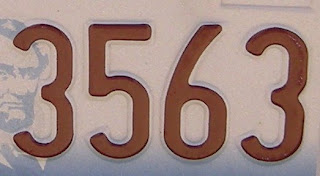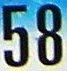## Friday, January 29, 2010

### 5497

5497 = 23 x 239.

5497 is 41241 in base 6, 7477 in base 9, and 2009 in base 14.

5497 is the number of lattice points within a sphere of radius 12.

5497 is a semiprime (product of two primes) such that 239 mod 23 = 9.NGC 5497 is a galaxy in the constellation Bootes.

## Thursday, January 28, 2010

### 4444

4444 = 22 x 11 x 101.

4444 is a number that cannot be written as a sum of three squares.

121 × (1 + 2 + 1) = 484 = 222
12,321 × (1 + 2 + 3 + 2 + 1) = 110,889 = 3332
12,34,321 × (1 + 2 + 3 + 4 + 3 + 2 + 1) = 19,749,136 = 4,4442

4444 is 32324 in base 6 and 15646 in base 7.The classic "four fours" problem states: "Given no more than four instances of the digit 4, represent all integers using a finite number of mathematical symbols and operators in common use."

Source: Numeropedia

## Wednesday, January 27, 2010

### 3563

3563 = 7 x 509.

3563 is a semiprime (product of two primes) such that 509 mod 7 = 5.

3563 is a term in the sequence created by starting with 1, then repeatedly reversing the digits and adding 76 to get the next term.NGC 3563 is a galaxy in the constellation Leo.

## Tuesday, January 26, 2010

### 2051

2051 = 7 x 293.

2051 is the sum of five positive fifth powers: 2051 = 15 + 15 + 15 + 45 + 45.

2051 is an odd number with exactly three 1s in its binary expansion: 100000000011.

A total lunar eclipse will occur on Oct. 19, 2051, as the moon passes through the center of Earth's shadow.

## Monday, January 25, 2010

### 1116

1116 = 22 x 32 x 31.

1116 is a Zuckerman number: a number divisible by the product of its digits (6).

1116 is the number of 8-abolos, polyominos consisting of eight isosceles right triangles joined along edges of the same length.1116 is 13431 in base 5 and 5100 in base 6.

1116 is a number that cannot be written as a sum of three squares.1116 Catriona was an asteroid discovered in 1929.

## Friday, January 22, 2010

### 203

203 = 7 x 29.

203 is the sixth Bell number.

2032 + 2030 + 2033 is a prime number.

The square of 203 is one of three squares that use the same digits: 1482 = 21904, 2032 = 41209, and 3022 = 91204.

203 is 21112 in base 3, 535 in base 6, and 313 in base 8.

The telephone area code 203 covers the southwestern part of Connecticut.

## Thursday, January 21, 2010

### 112

112 = 24 x 7.

112 is the difference between two squares: 112 = 112 - 32.

112 is a number that cannot be written as a sum of three squares.

112 is 11011 in base 3, 1300 in base 4, 422 in base 5, 220 in base 7, 88 in base 13, 80 in base 14, 77 in base 15, and 70 in base 16.

112 is the maximum number of regions into which 11 circles can divide a plane.

There are 112 pounds in one hundredweight (cwt). In the United States, a short hundredweight is 100 pounds.

## Wednesday, January 20, 2010

### 46

46 = 2 x 23.

46 = 10 + 11 + 12 + 13.

46 has a unique representation as a sum of three squares: 46 = 12 + 32 + 62.

46 is the number of integer partitions of 18 into distinct parts.

46 is the largest even integer for which there is no pair of abundant numbers that add up to it.Human beings have 46 chromosomes in every cell of their body.

## Tuesday, January 19, 2010

### 3000

3000 = 23 x 3 x 53.

3000 is MMM, the largest palindrome in Roman numerals.

3000 is 101110111000 in base 2, 232320 in base 4, 44000 in base 5, 2288 in base 11, 1144 in base 14, and 550 in base 24.

3000 is the number of symmetric arrangements of seven non-attacking queens on a 7 x 7 chessboard.

3000 is the smallest number requiring more than 12 letters to write in the English language. Three thousand uses 13 letters.

## Friday, January 15, 2010

### 915

915 = 3 x 5 x 61.

915 is a Smith number. The sum of its digits equals the sum of the digits of its prime factors: 9 + 1 + 5 = 3 + 5 + 6 + 1 = 15.

915 is 4123 in base 6, 555 in base 13, and 393 in base 16.

915 is the number of primes less that 104 that do not contain the digit 8.915 is a telephone area code in Texas for the area around El Paso.

## Thursday, January 14, 2010

### 898

898 = 2 x 449.

898 has a representation as a sum of two squares: 898 = 132 + 272.

898 is a member of the Fibonacci-type sequence starting with 2 and 5.

898 is 747 in base 11.ISO 898 is an international standard that defines mechanical and physical properties for metric fasteners.

## Wednesday, January 13, 2010

### 720

720 = 24 x 32 x 5.

720 is the smallest number with 30 divisors.

720 has a representation as a sum of two squares: 720 = 122 + 242.

720 = 6! It is the largest factorial to contain all different digits.

720 is the smallest number n where either n or n + 1 is divisible by the numbers from 1 to 10.The epic poem Beowulf was composed in the year 720.

## Tuesday, January 12, 2010

### 698

698 = 2 x 349.

698 has a representation as a sum of two squares: 698 = 132 + 232.

698 is 221212 in base 3, 22322 in base 4, and 585 in base 11.

698 is the smallest of three consecutive integers divisible by three consecutive primes respectively: 698 = 2 x 349, 699 = 3 x 233, 700 = 5 x 140.NGC 698 is a galaxy in the constellation Fornax.

## Monday, January 11, 2010

### 512

512 = 29.

512 has a representation as a sum of two squares: 512 = 162 + 162.

512 is the number of integer partitions of 34 into distinct parts.

512 is the smallest integer (except 1) that is the cube of the sum of its digits: 512 = (5 + 1 + 2)3.

512 is 1000000000 in base 2, 20000 in base 4, 1331 in base 7, 1000 in base 8, 242 in base 15, and 200 in base 16.512 is the three-digit start in the Dewey Decimal Classification System of call numbers for books in algebra and number theory.

## Friday, January 8, 2010

### 488

488 = 23 x 61.

488 has a representation as a sum of two squares: 488 = 22 + 222.

4883 = 116,214,272 = (1 - 1 + 62 + 1 + 427 - 2)3.

488 is 200002 in base 3, 404 in base 11, and 148 in base 20.IEEE-488 is a short-range digital communications bus specification, originally created for use with automated test equipment.

Source: Numeropedia

## Thursday, January 7, 2010

### 323

323 = 17 x 19.

323 is the smallest palindrome that is the product of a pair of twin primes.

323 is the smallest palindrome that is not divisible by any of its digits.

323 is the 8th Motzkin number. These numbers enumerate various combinatorial objects.

323 Brucia was the first asteroid to be discovered by the use of astrophotography.

## Wednesday, January 6, 2010

### 293

293 is a prime number.

293 is a Sophie Germain prime because 2 x 293 + 1 = 587, which is also a prime.

293 has a representation as a sum of two squares: 293= 22 + 172.

293 is the hypotenuse of a primitive Pythagorean triple: 2932 = 682 + 2852.

293 Brasilia is a large asteroid discovered in 1893.

## Tuesday, January 5, 2010

### 103

103 is a prime number.

103 is the smallest three-digit prime with distinct digits.

101 and 103 form a twin prime pair.

103 is a number that cannot be written as a sum of three squares.

103 is the total number of prime numbers that can be displayed on a 12-hour digital clock.

The play Proof, by David Auburn, revolves around a mysterious proof involving prime numbers, left behind in 103 notebooks by a young woman's brilliant father.

## Monday, January 4, 2010

### 58

58 = 2 x 29.

58 has a representation as a sum of two squares: 58 = 32 + 72.

58 is the maximum number of regions into which eight circles divide the plane.

58 is the smallest Smith number with a prime sum of digits. A Smith number is a composite number the sum of whose digits is the sum of the digits of its prime factors: 5 + 8 = 2 + 2 + 9 = 13.

58 is the number of commutative semigroups of order 4.The chemical element cerium has the atomic number 58.

Source: Number Gossip